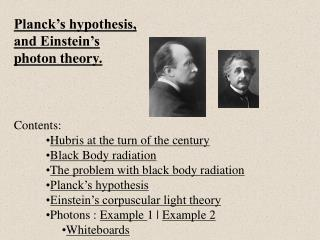# Planck’s hypothesis, and Einstein’s photon theory. Contents: Hubris at the turn of the century - PowerPoint PPT PresentationDownload PresentationPlanck’s hypothesis, and Einstein’s photon theory. Contents: Hubris at the turn of the century

Planck’s hypothesis, and Einstein’s photon theory. Contents: Hubris at the turn of the centuryDownload Presentation## Planck’s hypothesis, and Einstein’s photon theory. Contents: Hubris at the turn of the century

- - - - - - - - - - - - - - - - - - - - - - - - - - - E N D - - - - - - - - - - - - - - - - - - - - - - - - - - -
##### Presentation Transcript

1. Planck’s hypothesis, • and Einstein’s • photon theory. • Contents: • Hubris at the turn of the century • Black Body radiation • The problem with black body radiation • Planck’s hypothesis • Einstein’s corpuscular light theory • Photons : Example 1 | Example 2 • Whiteboards

2. Black Body radiation Radiation given off by hot stuff What BBR is – demo Note peak – hotter is bluer The end of physics Planck’s problem The solution Steps vs. ramp Vibrations are “auto tuned” TOC

3. Einstein’s photon theory • Einstein: (Quantum jump) • Photons = corpuscular theory • E = hf • E = Photon energy (Joules) • h = Planck’s constant = 6.626 x 10-34 Js • f = frequency of oscillations (Hz, s-1) • v = f • v = c = speed of light = 3.00 x 108 m/s • f = frequency (Hz) •  = wavelength (m) • Ex. 1: Energy of a 460 nm photon? • Ex. 2: Wavelength of a 13.6 eV photon? TOC

4. E = hf v = f E = Photon energy (Joules) v = c = speed of light = 3.00 x 108 m/s f = frequency (Hz)  = wavelength (m) Example 1: What is the energy of a 460. nm photon? v = f, c = f, f = c/ E = hf = hc/ = (6.626E-34 Js)(3.00E8 m/s)/(460E-9m) = 4.3213E-19 J = (4.3213E-19 J)/(1.602E-19 J/eV) = 2.70 eV TOC

5. E = hf v = f E = Photon energy (Joules) v = c = speed of light = 3.00 x 108 m/s f = frequency (Hz)  = wavelength (m) Example 2: A photon has an energy of 13.6 eV. What is its wavelength? (Remember V = W/q, and 1 eV is one electron moved through 1 volt) E (in Joules) = (13.6 eV)(1.602 x 10-19 J/eV) = 2.17872E-18 J c = f,  = c/f E = hf, f = E/h  = c/f = hc/E = (6.626E-34 Js)(3.00E8 m/s)/(2.17872E-18 J) = 9.12371E-08 m = 91.2 nm (1 nm = 1x10-9 m) TOC

6. Whiteboards: Photons 1 | 2 | 3 | 4 TOC

7. What is the energy (in J) of a photon with a frequency of 6.58 x 1014 Hz? E = hf = (6.626 x 10-34 Js)(6.58 x 1014 s-1) = 4.36 x 10-19 J W 4.36 x 10-19 J

8. What is the wavelength of a photon with an energy of 5.45 x 10-18 J? E = hf f = E/h = (5.45 x 10-18 J)/(6.626 x 10-34 Js) = 8.23 x 1015 Hz v = f,  = v/f = (3.00 x 108 m/s)/(8.23 x 1015 Hz) = 3.65E-8 m = 36.5 nm W 36.5 nm

9. What is the energy (in eV) of a 314 nm photon? (2) E = hf = hc/ 1eV = 1.602 x 10-19 J E = hc/ = (6.626 x 10-34 Js)(3.00 x 108 m/s)/(314 x 10-9 m) = 6.33 x 10-19 J E = (6.33 x 10-19 J)/(1.602 x 10-19 J/eV) = 3.95 eV W 6.33 x 10-19 J, 3.95 eV

10. A photon has an energy of 6.02 eV. What is its wavelength? (answer in nm) (2) E = hf = hc/ 1eV = 1.602 x 10-19 J E = (6.02 eV)(1.602 x 10-19 J/eV) = 9.64 x 10-19 J E = hc/,  = hc/E = (6.626 x 10-34 Js)(3.00 x 108 m/s)/(9.64 x 10-19 J) = 2.06 x 10-7 m = 206 nm (nm = 1 x 10-9 m) W 206 nm

11. How many photons per second stream from a 620. nm, 300. mW laser? (2) E = hf = hc/ In one second, a 300 mW laser emits photons with a total energy of .300 J E = hc/, = (6.626 x 10-34 Js)(3.00 x 108 m/s)/(620. x 10-9 m) = 3.20613 x 10-19 J (per photon) # photons = (.300 J/s)/(3.20613 x 10-19 J/photon) = 9.36 x 1017 photons/sec Hey, that’s a lot! W 9.36 x 1017 photons/sec

12. small  = blue big  = red small = dim big = bright Light Waves Photons Wavelength Changes Energy per photon changes E = hf Radio Waves vs. X-rays Color Amplitude Changes # of photons changes many = bright few = dim CCD Devices, High speed film Brightness